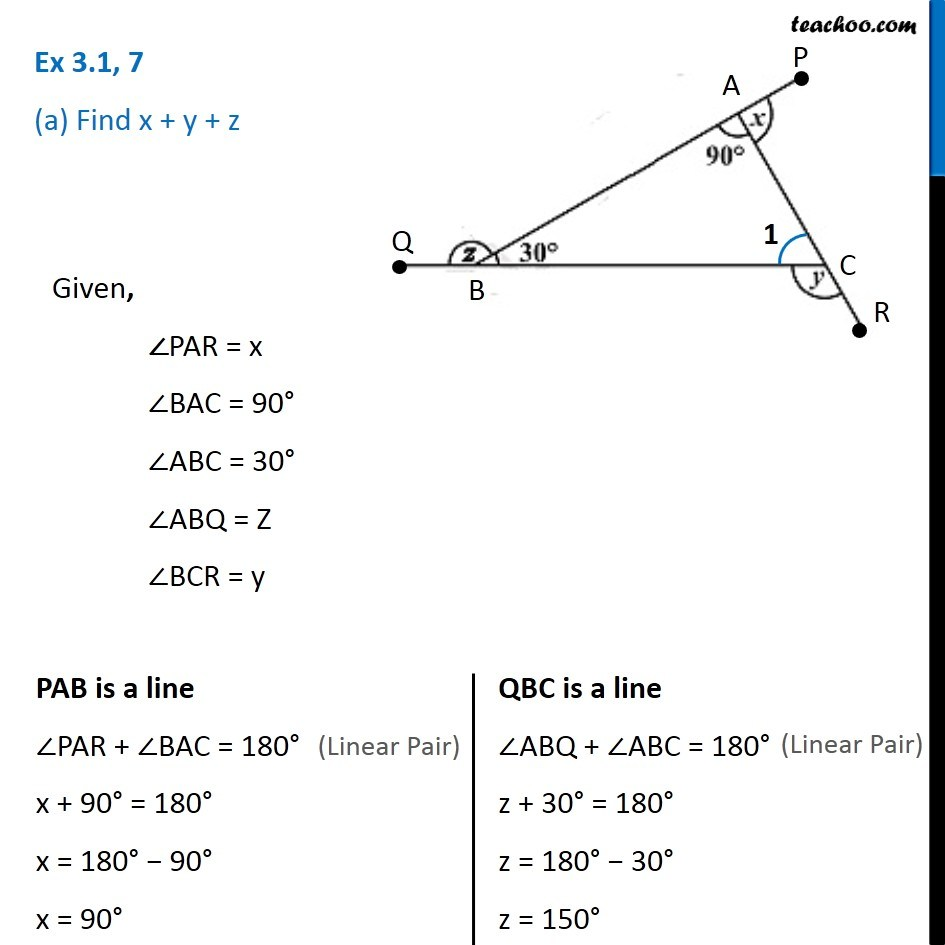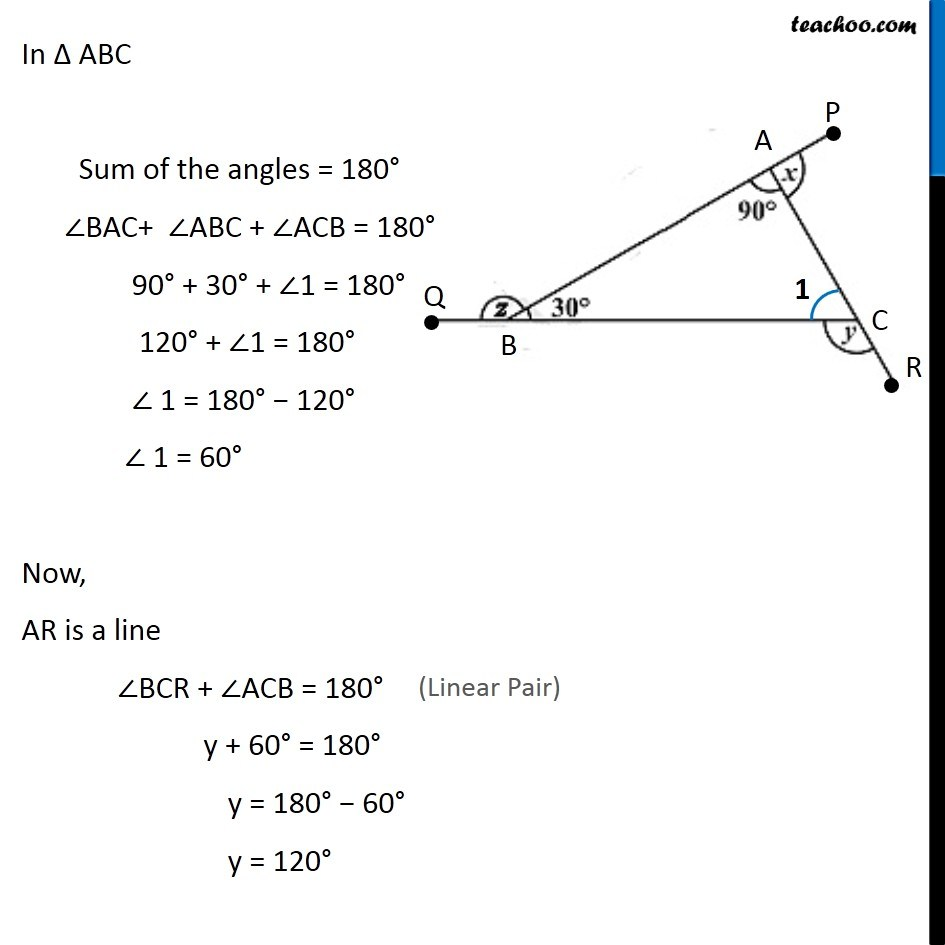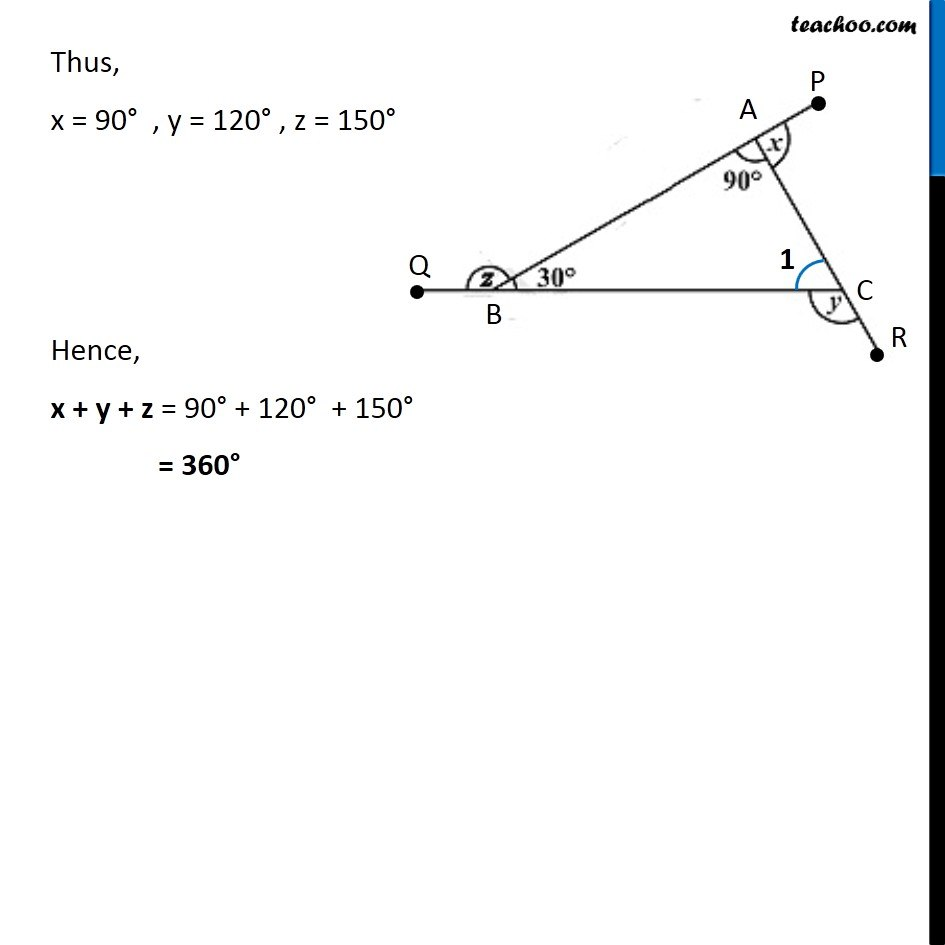Ex 3.1

Chapter 3 Class 8 Understanding Quadrilaterals
Serial order wiseLearn in your speed, with individual attention - Teachoo Maths 1-on-1 Class

### Transcript

Ex 3.1, 7 (a) Find x + y + zGiven, ∠PAR = x ∠BAC = 90° ∠ABC = 30° ∠ABQ = Z ∠BCR = y PAB is a line ∠PAR + ∠BAC = 180° x + 90° = 180° x = 180° − 90° x = 90° QBC is a line ∠ABQ + ∠ABC = 180° z + 30° = 180° z = 180° − 30° z = 150° In ∆ ABC Sum of the angles = 180° ∠BAC+ ∠ABC + ∠ACB = 180° 90° + 30° + ∠1 = 180° 120° + ∠1 = 180° ∠ 1 = 180° − 120° ∠ 1 = 60° Now, AR is a line ∠BCR + ∠ACB = 180° y + 60° = 180° y = 180° − 60° y = 120° Thus, x = 90° , y = 120° , z = 150° Hence, x + y + z = 90° + 120° + 150° = 360°